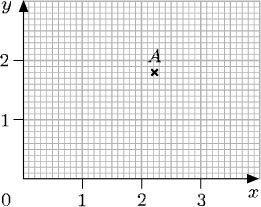The basic SI unit for mass is the kilogram, symbol kg

The tonne (t) which is equivalent to 1000 kg and is a metric unit is often used alongside the SI units.

The animation below illustrates how to convert between the most commonly used units of mass, the metric tonne (t); the kilogram (kg); the gram (g); the milligram (mg) and the microgram (μg).

Author(s): The Open University

## Activity 17

A person's height is given as 1.65 m. What is this in centimetres?

1.65 m is 165 cm (multiply by 100).

Author(s): The Open University

A great advantage of the metric system of units is that conversion between units within the system is particularly easy. For example, ‘£1 is worth 100p’ is converting one pound into pence. To convert pounds to pence, you multiply by 100. So £2 is 200p, and £2.63 is 263p. (Remember that to multiply by 100, you move the digits two places to the left in the place value table.)

To convert from pence to pounds, you need to reverse this process, i.e. to divide by 100 (moving the
Author(s): The Open University

## Activity 15

Suggest appropriate units for each of the following:

• (a) the age of the kitten when it is weaned;

• (b) the distance between one train station and the
Author(s): The Open University

It is important to choose appropriate units, both to have a sense of the size you are talking about and also to avoid having to deal with very large or very small numbers.

For example using the UK decimal system, where £1 = 100p (one pound is equivalent to 100 pence), you would probably use pence for prices less than a pound: 50p rather than £0.50 and pounds for larger amounts, e.g. £2.50 instead of 250p.

For distance, mass and liquid measures, the metric system of units
Author(s): The Open University

Numbers are used to count things (such as 54 sheep in a field) or, together with units of measurement, to quantify something (such as a 2-litre water bottle or a 3-year waiting list for an operation). This section reminds you of some units of measurement and considers appropriate units to use. We also need to make comparisons between quantities given in different units.

Author(s): The Open University

## Activity 14

Contour lines on a map show all the points at a given height above sea level. The lines are drawn for each height at 50-metre intervals, and points below sea level are shown by negative heights. The diagra
Author(s): The Open University

Numbers can be positive or negative, i.e. greater than or less than zero. Negative numbers have several uses; for example, to measure temperatures below zero, such as 3°C (‘minus 3 degrees Celsius’). They are also used to represent debts and overdrawn accounts: a bank balance of £84.33 means ‘overdrawn by £84.33’.

Negative numbers are shown on the number line to the left of 0. The animation below shows 8, 7,
Author(s): The Open University

A fraction is written as one number over another (such as) and means the top number divided by the bottom number. The top number, 3, is called the numerator and the bottom number, 10, is call
Author(s): The Open University

## Activity 6

What is 370.76 grams in kilograms? There are 1000 grams in a kilogram.

370.76 ÷ 1000 = 0.370 76.

So 370.76 g = 0.370
Author(s): The Open University

What do the digits to the right of the decimal point mean? The first column to the right of the decimal point is obtained by dividing the units by 10; this is the ‘tenths’ column. Similarly the next column contains the hundredths, and so on. So here is the extended place value table:Author(s): The Open University

## Activity 2

Write each of the following three numbers in numerals and then place them in ascending order:

• eight hundred and eight thousand

• two million and twenty
Author(s): The Open University

## Activity 1

Can you answer the following questions:

• (a) Write ‘twenty thousand one hundred and forty-four’ as a number.

• (b) Say (or write) the number 31 002
Author(s): The Open University

After studying this course, you should be able to:

• write whole numbers and decimals in place-value columns and compare their sizes

• multiply and divide whole numbers and decimals by 10, 100, 1000 and so on

• indicate given fractions on a diagram and find equivalent fractions for a given fraction

• mark numbers on a number line

• choose appropriate units for a given purpose.

Author(s): The Open University

The content acknowledged below is Proprietary (see terms and conditions) and is made available under a Creative Commons Attribution-NonCommercial-ShareAlike 4.0 Licence

Grateful acknowledgement is made to the following sources for permission to reproduce material in this course:<
Author(s): The Open University## Study another free course

There are more than 800 courses on OpenLearn for you to
Author(s): The Open University

Now try the quiz and see if there are any areas you need to work on.

Author(s): The Open University

## Activity 22

Write down the coordinates of the point P on each of the graphs below and interpret these coordinates in terms of the labels on the axes.

Author(s): The Open University

## Activity 20

Look at the diagram below and answer the following questions:

• (a) Write down the coordinates of the points P, Q, R, S and T.

Author(s): The Open University

Where necessary, the coordinates of a point can be specified by using decimals or fractions. However, locating points whose coordinates are not whole numbers requires more precision when reading along the axes. For example, here the coordinates of the point A are (2.2, 1.8).Author(s): The Open University